# Introduction to Data Structures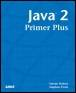Java™ 2 Primer PlusBy Steven Haines, Steve Potts Table of Contents Chapter 11.  Collection Classes

Data structures at their core are nothing more than containers that hold objects and provide mechanisms to add objects, remove objects, and find objects. After careful consideration, there are a multitude of different implementations of containers, each serving a very specific purpose. Some are very fast at inserting objects, whereas others are slow inserting objects, but very fast at finding objects. The key is to identify how you will be using the data stored in these collections.

The following sections describe the more common data structures and explain how each addresses the issues of inserting objects, removing objects, and finding objects.

#### The Array Data Structure

The simplest data structure is probably the array. An array is a fixed-size container that provides direct access to each element in the container through a 0-based index; there is no sorting of elements or management functions to add a remove objects. Figure 11.1 shows what an array looks like in memory.

##### Figure 11.1. Sample array.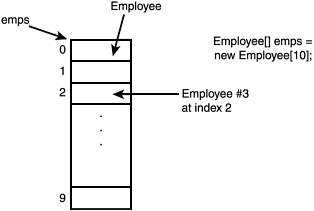In memory an array is a sequential list of objects, one following another, with each cell the exact size to hold the object type (or in the case of the true object and not a primitive type, the object reference).

An array is created in Java as follows:

` // Create an array that will hold 10 employees:  Employee[] employees = new Employee[ 10 ]; `

employees is of the type Employee[] that represents an array of the type Employee. It is initialized to hold 10 Employee instances using the keyword new and specifying the size within the brackets. At this point employees has allocated enough memory to hold 10 Employee references.

To add a new employee to the beginning of the array, you must first create an instance of an Employee and assign it to the first elements in the employees array, for example:

` // Set the first employee to be "Steve"  Employee steve = new Employee( "Steve" );  employees[ 0 ] = steve; `

Array elements are accessed via a 0-based index, so the first elements in the array have index 0, the second elements of the array have index 1, and so on. So, in this case we created an instance of the hypothetical Employee class passing it to a name, and then we assign that instance to the first elements of the employees array.

To search through an array of an element there is no simple mechanism to instantly find the element you are looking for, you must instead use a brute-force traversal of every element in the array. For example:

` // Find Steve:  for( int index=0; index<employees.length; index++ ) {    if( employees[ index ].getName().equalsIgnoreCase( "Steve" ) ) {      System.out.println( "Steve is employee at index: " + index );      break;    }  } `

Here we start at an index of 0 and iterate through the list of employees until we reach the index employees.length. Arrays define a property named length that returns the size of the array, which in this example would be 10. Thus, the worst-case time required to locate an object in an array can be equal to accessing and comparing every element in the array.

Removing an element from an array after you know the index of the element to remove is as simple as overwriting the element or assigning it the null value. For example:

` // Remove Steve:  employees[ 0 ] = null; `

Arrays provide a simple mechanism for grouping similar objects in a sequential fashion, but they do not provide any inherent functionality to add, remove, or search for an object. When you use arrays, the burden of these tasks is on you.

Arrays are very simple and because of this simplicity have a set of strong limitations:

• An array has a fixed size, meaning that if you want to add an additional element to a full array you cannot.

• Upon the deletion of an element from the array, the array has no inheritance mechanism to shift the existing elements up in the array to fill in the missing gap; this means that your search implementation must search through meaningful data as well as null data.

• The burden of defining all functionality, except obtaining the length of an array, is on the developer.

#### The Linked List Data Structure

A linked list solves the fixed-length problem with arrays and has an interesting approach to inserting and removing objects. A linked list is a set of nodes in which each node contains an object and a reference to the next node in the linked list (see Figure 11.2).

##### Figure 11.2. A linked list.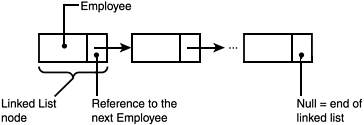Figure 11.2 shows that the linked list node contains an Employee (or a reference to Employee rather), and then a reference to the next node in the linked list. The end of a linked list is signified by a null reference to the next node field. Because each node is linked to the next node the length of the linked list can be infinite.

Adding a new element to the end of the list is a simple matter of assigning the next node reference in the end of the list (often referred to as the tail of the list) to the new element. Then, assigning the next node reference in the new elements to null (see Figure 11.3).

##### Figure 11.3. Adding an element to a linked list.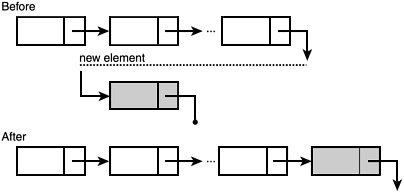Removing an object from a linked list is a more complicated procedure, but not significantly. After you identify the node to remove, it is a simple matter of reassigning the next node element of the previous node to the next node element of the node being removed. This is much better illustrated graphically, so refer to Figure 11.4.

##### Figure 11.4. Removing an element from a linked list.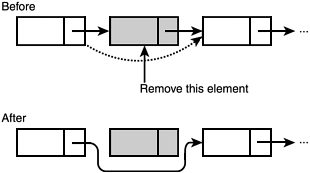Finally, inserting an element into a linked list involves breaking the next node reference at the insertion point, referencing it to the new node, and assigning the next node element of the new node to the next node in the list. Again, this is much better illustrated graphically, so refer to Figure 11.5.

##### Figure 11.5. Inserting an element in a linked list.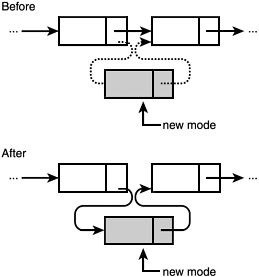Technically, this is how linked lists are implemented, but luckily Java has already implemented a linked list for you through its java.util.LinkedList class. But, before delving into the intricacies of using that class let's step back a moment and analyze the performance of using linked lists.

The insertion of an element into a linked list involves

• Creating a new linked list node

• Reassigning two next element references

Thus, the operation is quite fast.

The removal of an element from a linked list involves the deletion of a linked list node and then reassigning one next element reference. Thus, this operation is quite fast.

Searching for an element in a linked list, however, is a very slow operation and can involve examining each element in the linked list. There is no random access of elements in the list and common implementations can only reference the beginning of the list (referred to as the head) and the end of the list (referred to as the tail). The search for an element involves the examination of the node, then following its next element reference to the next node and examining it, and so on. Some implementations attempt to optimize this by sorting the elements in the linked list and providing links to nodes in both directions; this is referred to as a doubly linked list. But still the search is slow (see Figure 11.6).

##### Figure 11.6. A doubly linked list.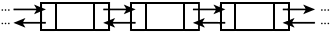Linked lists are, therefore, good when you need to rapidly insert and/or remove items from a list, but not when you need to search for objects or display different sorted orders of those objects.

#### The Stack Data Structure

A stack is a data structure that can be described by comparing it to a stack of books: you place a book on a table, then another on top of it, and another on top of that, and so on. After you have this stack of books there are a limited list of things you can do with it:

• You can add another book on top of it, but not randomly in the stack.

• You can look at the top book on the stack, but you cannot see the books below the top one.

• You can take the book off the top of the stack, but you cannot take one from the middle.

A stack, as a data structure, has the same functionality, but with some key terminology:

• You can "push" an object onto the top of the stack

• You can "peek" at the object on the top of the stack

• You can "pop" an object off the top of the stack

Figure 11.7 shows this graphically.

##### Figure 11.7. A stack.It turns out that stacks work very well for mathematical operations and for developing computer compilers, but aside from that they offer very poor performance for the operations we have been reviewing. Adding an item to the stack is very fast, but inserting an item into the stack involves popping all the items off the stack to the point of insertion, pushing the new item onto the stack, and then pushing the popped objects back on; therefore, it is very slow. Removing an object from the stack similarly requires popping all items from the stack to the deletion point, and then pushing the popped items back onto the stack. Finally, searching for an item requires popping each item off the stack until the desired object is found, and then repushing all objects back on the stack.

From this discussion you can see that a stack is not good to use except for very specific functions.

#### The Queue Data Structure

A queue is a data structure that can be likened to a line in a supermarket: Everyone gets in line and unless someone is very rude, the person at the front of the line is waited on first, and then each subsequent person is waited on in order. Under normal circumstances a person cannot go to the front of the line, or anywhere in the middle of the line, he must go to the back of the line and wait his turn.

A queue, as a data structure, follows a similar pattern:

• An item can be enqueued, or added, to the end of the queue.

• An item can be dequeued, or removed, from the front of the queue.

Figure 11.8 displays this graphically.

##### Figure 11.8. A queue.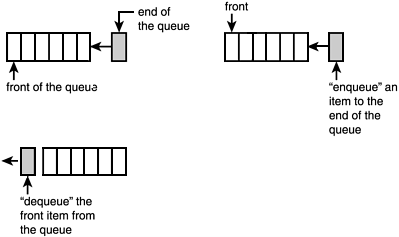Queues are very good when you need to service requests as they arrive and are very popular when handling events and handling messages, but you can extrapolate out its functionality to see that it does not fit very cleanly into our insert, remove, and search operations.

#### The Hash Table Data Structure

A hash table is a data structure that computes a numerical value for an item, and then references that item by that numerical value in a table. The table has two columns: the hash code and the item itself (see Figure 11.9).

##### Figure 11.9. A hash table.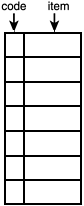Insertion into the table involves computing a "hash code" for the object, and then inserting the object at that index of the hash table. Finding an object is very simple, compute the hash code, and then it is a direct lookup, you do not need to traverse all the items in the hash table to find the one you are looking for, as with arrays. This works great if you can ensure that you can always compute unique hash codes in the range supported by the hash table. But, if two objects compute to the same hash code, then you have a collision. Hash tables are usually defined with a size about 20% greater than the largest amount of data they can hold to avoid collisions, but collisions can occur nonetheless.

When two objects collide in the hash table, the collision must be resolved. Different implementations of hash code algorithms do different things, ranging from the simple "move to the next slot until you find an opening," to a more complex computation of another hash code value. Figure 11.10 shows a simple example of hash code collision resolution.

##### Figure 11.10. Hash code collision.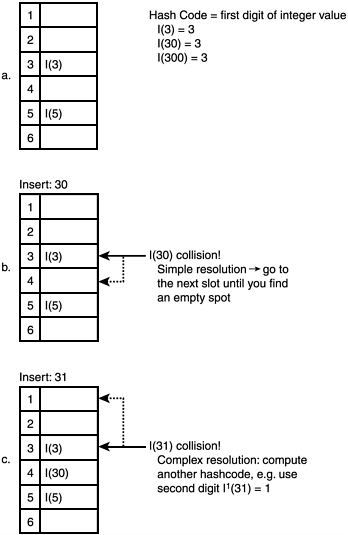In this example, we use Integer objects and define the hash code algorithm to use the leading number of the Integer as the index into the hash table. Thus, the hash code of 3 would be 3, and the hash code for 30 would be 3. Figure 11.10 shows the simple resolution of moving to the next open slot. Figure 11.10 also shows the insertion of 31, which resolves to 3, and would require the examination of slot 4, then 5, and then, finally, 6. But instead this implementation uses another hash algorithm to compute a second hash code; in this case it uses the second digit of the Integer value, so I'( 31 ) would be 1. Most competent hash code algorithms use a resolution algorithm that, as long as the size of the hash table is sufficient, guarantees that at most two resolution hash codes will be computed before finding a unique slot.

The implementation of hash code algorithms is beyond the scope of this book, but I encourage you to do more research if you are interested.

#### The Tree Data Structure

Trees provide a very sophisticated mechanism for storing data; each node in a tree assumes a role: it is either the parent to other nodes, a child to a node, or both. Figure 11.11 shows an example of a tree.

##### Figure 11.11. A visual depiction of a tree.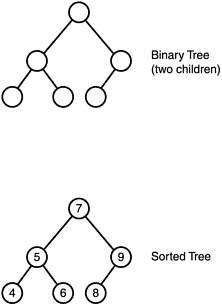In computer science there are two types of trees: binary trees and B-Trees. A binary tree restricts each node to having at most two children, whereas a B-Tree removes this restriction. For the purposes of this discussion when referring to trees I will be talking about binary trees.

From Figure 11.11 you can see that node values are placed in a tree in a very specific way. The root of the binary tree in Figure 11.11 has the value 7. The values of all nodes to the left of the root have a value less than 7, whereas all nodes to the right have a value greater than 7. This same relationship is true of every node in a tree: The node with a value 5 has a child to its left with the value 4 and a child to its right with the value 6.

Searching a tree for a node after you know the node's value is very fast and almost a trivial operation. Figure 11.12 shows an example of locating the node with value 6.

##### Figure 11.12. Searching a binary tree for the value 6.The search algorithm first looks at the root and sees that the value is 7, which is greater than 6, so it traverses the left subtree. Next, it looks at the root of this subtree and sees that the value is 5, which is less than 6, so it traverses the right subtree. On the third search it finds the node for which it's looking, and the search is complete.

The nature of trees exhibits this sorted property and insertion algorithms ensure that the tree stays balanced. A balanced tree has the property that each subtree to the left of the node has approximately the same number of elements as the subtree to the right of the node. Because this is enforced, the average time to find a node in the tree is the natural logarithm of the number of elements in the tree. So, for example, if the tree had 25 elements, the average number of nodes that have to be examined before finding any elements in the tree is ln(25) = 3.22. If you extrapolate this to hundreds of nodes, or even thousands of nodes, the search time has incredible performance.

Inserting the item into a tree is not quite as simple of an operation. The property that gives trees such rapid search capabilities is a direct result of insertion and removal functionality keeping the tree balanced. This means that when you insert an element into the tree you might have to adjust the very nature of the tree itself (see Figure 11.13).

##### Figure 11.13. Inserting a 7 into the tree.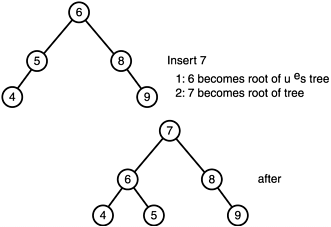From Figure 11.13 you can see that inserting the 7 node requires restructuring the architecture of the tree. The algorithm displayed in Figure 11.13 required that the 7 become the root of the tree and, thus, the current root had to be shifted down and its children moved around to keep it balanced. The insertion operation can be much slower than the insertion into other data structures such as a linked list, and it is most appropriate when insertions and removals will be minimized and searches will be frequent.

Figure 11.14 shows an example of removing an object from the tree.

##### Figure 11.14. Removing a node from the tree.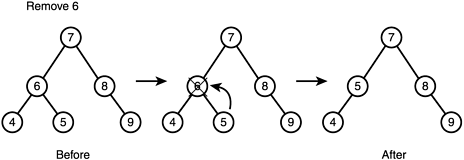You can see that removing the 6 node requires the 5 node to shift up and take its place. A more complicated example might be removing the 7 node, or root node, from the tree. The end result is that to maintain the balance of the tree another node must be elevated and assume the root responsibility. (This can be a direct child of the root node, or it can be a leaf somewhere in the tree that is the optimal choice for balancing the tree.)

Algorithms for inserting or removing objects from the tree is beyond the scope of this chapter, but further study is encouraged. For the purposes of this discussion, you should understand the operations that must take place and the associated cost of those operations.

To summarize the use of a tree, consider the nature of your data and how will be manipulated. If you need to rapidly add and remove objects from your data structure, a tree is not your best solution. But, if you are going to sparingly add and remove objects from the data structure, and need to search the data structure frequently, a tree is your best bet.Top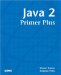Java 2 Primer Plus
ISBN: 0672324156
EAN: 2147483647
Year: 2001
Pages: 332

Similar book on Amazon

flylib.com © 2008-2017.
If you may any questions please contact us: flylib@qtcs.net